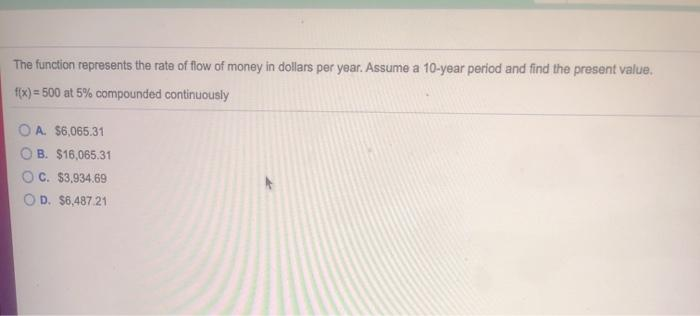### Create an Account

Home / Questions / The function represents the rate of flow of money in dollars per year. Assume a 10-year pe...

# The function represents the rate of flow of money in dollars per year. Assume a 10-year period and find the present value. f(x) = 500 at 5% compounded continuously O A. \$6,065.31 B. \$16,065.31 C. \$3,9

The function represents the rate of flow of money in dollars per year. Assume a 10-year period and find the present value. f(x) = 500 at 5% compounded continuously O A. \$6,065.31 B. \$16,065.31 C. \$3,934.69 D. \$6.487 21Apr 29 2021 View more View LessSubscribe To Get Solution